# Arcsine law

(diff) ← Older revision | Latest revision (diff) | Newer revision → (diff)

A limit theorem describing the fluctuations of a random walk on the real line, which results in an arcsine distribution or a generalized arcsine distribution. The following feature of a Brownian motion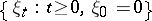was noted in 1939 by P. Lévy. Letbe the Lebesgue measure of the set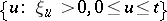or, in other words, the time spent by a Brownian particle on the positive semi-axis during the interval of time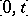. The ratiowill then have the arcsine distribution: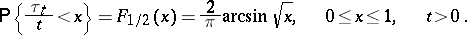It was subsequently noted  that a random walk with discrete time obeys the following arcsine law: Let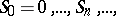be the successive locations in the random walk,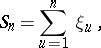whereare independent and identically distributed, let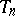be equal to the number of indicesamong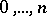for which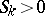, and let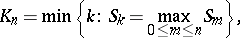then the relationships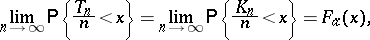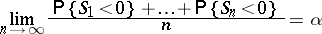are all satisfied or not satisfied at the same time; here,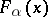foris the generalized arcsine distribution,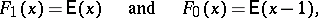whereif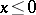andif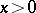.

The arcsine law in renewal theory states that forthe following equalities are valid: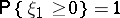and for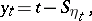whereis defined by the relation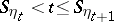,if and only if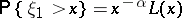for, whereis a function which is defined forand which has the property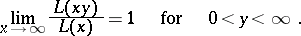There exists a close connection between the arcsine law in renewal theory and the arcsine law governing a random walk .

How to Cite This Entry:
Arcsine law. Encyclopedia of Mathematics. URL: http://encyclopediaofmath.org/index.php?title=Arcsine_law&oldid=18102
This article was adapted from an original article by B.A. Rogozin (originator), which appeared in Encyclopedia of Mathematics - ISBN 1402006098. See original article